# 3D Geometric ShapesHey guys! Welcome to this Mometrix video on 3D Geometric Shape Identification.

First, what we will do is take a look at what a 3D shape is, then we’ll look at the different properties of a 3D shape.

Let’s do a quick review. A 1D shape has a length. 2D only has length and a width.

So, what is a 3D shape? Well, 3D stands for three-dimensional; those three different dimensions are: length, width, and depth (or height) for a polyhedron, and base, radius, and height for a curved three-dimensional shape. A polyhedron is a three-dimensional shape with all flat faces. A curved three-dimensional shape would be like a sphere, cone, or cylinder. In this video, we will focus on polyhedrons.

Length refers to the measurement of something from one end of a segment, all the way to the other.

Width refers to the measurement of something from one side of a segment, to the other side.

Depth (or height) refers to the distance from the top of a segment to the bottom.

Now, let’s talk about the properties of a three-dimensional shape. Three-dimensional polyhedrons have faces, edges, and vertices.

A face refers to the surface of a three-dimensional shape. A cube has 6 faces.

Edge refers to the where two faces connect. A cube has twelve edges.

A vertex (or vertices when you are referring to more than one) are the corners, or where the edges come together. A cube has 8 vertices.

Understanding 3D shapes and their properties are very important, and will help you to do much more, like calculate volume.

I hope that this video over 3D geometric shape identification has been helpful. If you enjoyed it, then be sure to give us a thumbs up, and subscribe to our channel for further videos.

See you guys next time!

## Practice Questions

Question #1:

Which of the following is not a property of a three-dimensional shape?

Number of vertices

Number of angles

Number of faces

Number of edges

The three properties of a three-dimensional shape are the numbers of faces, edges, and vertices. Remember, the number of faces describes how many sides the shape has. The number of edges represents the amount of places where two faces meet (like a corner). The number of vertices describes how many locations on the shape are points where corners of faces touch.

Question #2:

How many faces does the diamond shown below have?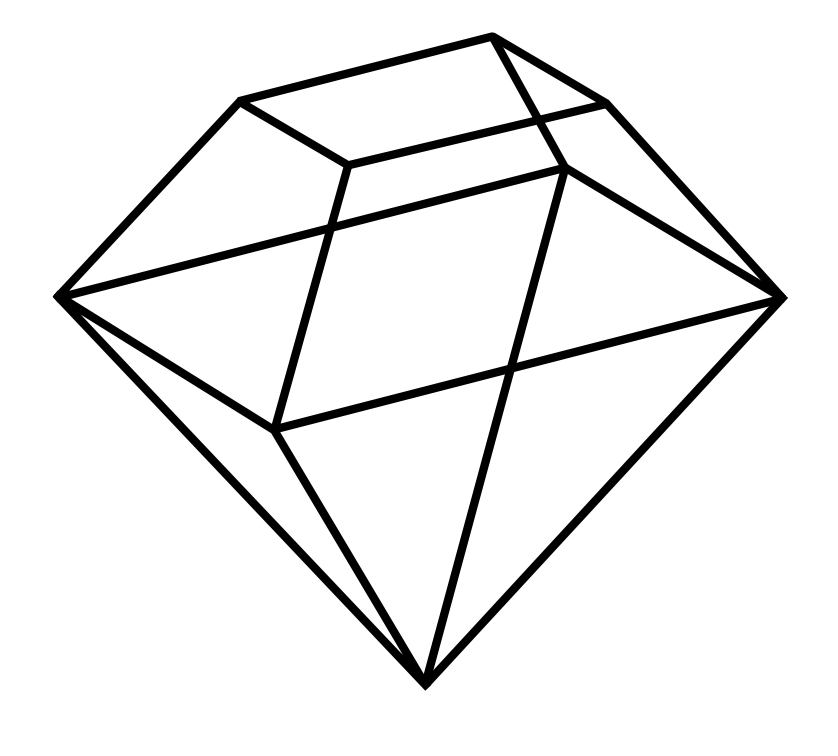8 faces

9 faces

10 faces

11 faces

The diamond shown has 9 faces; one on the very top, then four around it on the upper portion of the diamond, and four more composing the base.

Question #3:

How many edges does the square pyramid shown below have?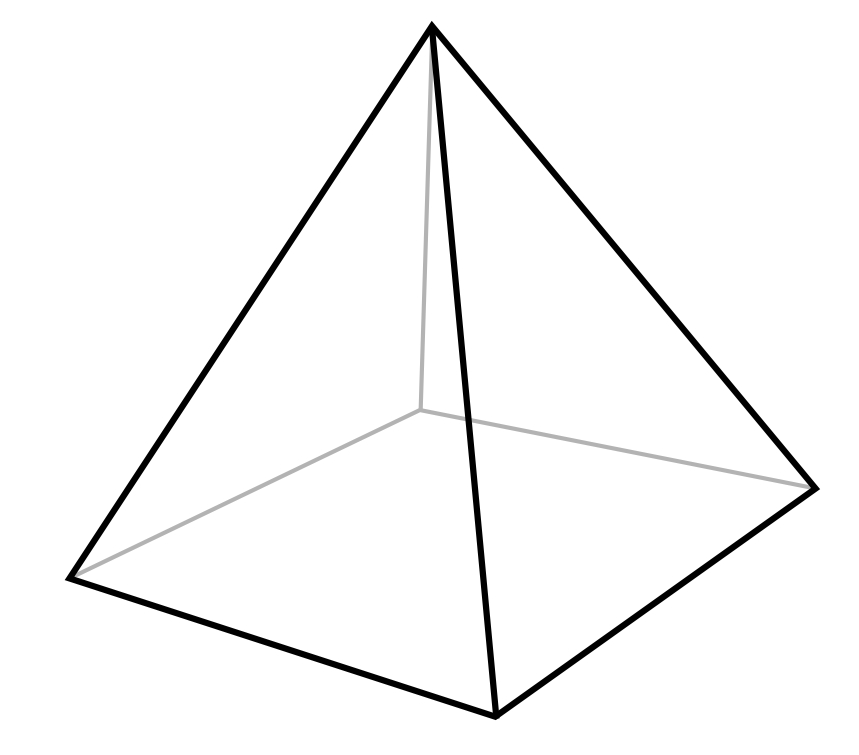5 edges

6 edges

7 edges

8 edges

Square pyramids have 8 edges; four of them outline the square base, while the other four point to and meet at the top.

Question #4:

Observe the following image of a triangular prism. How many vertices does it have?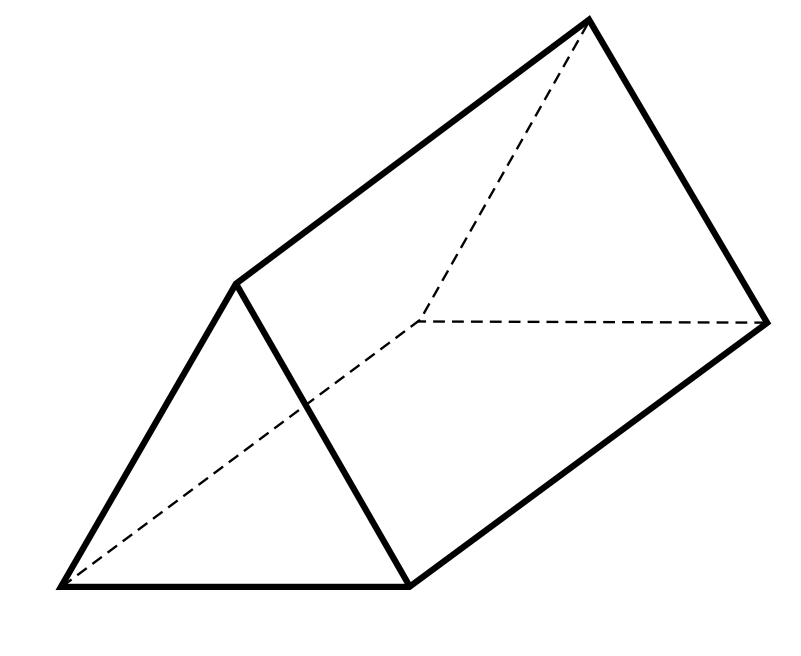3

5

6

9

Triangular prisms have six vertices, the points where the edges come together. Three are located at each end, on the points of the triangles forming either end of the prism.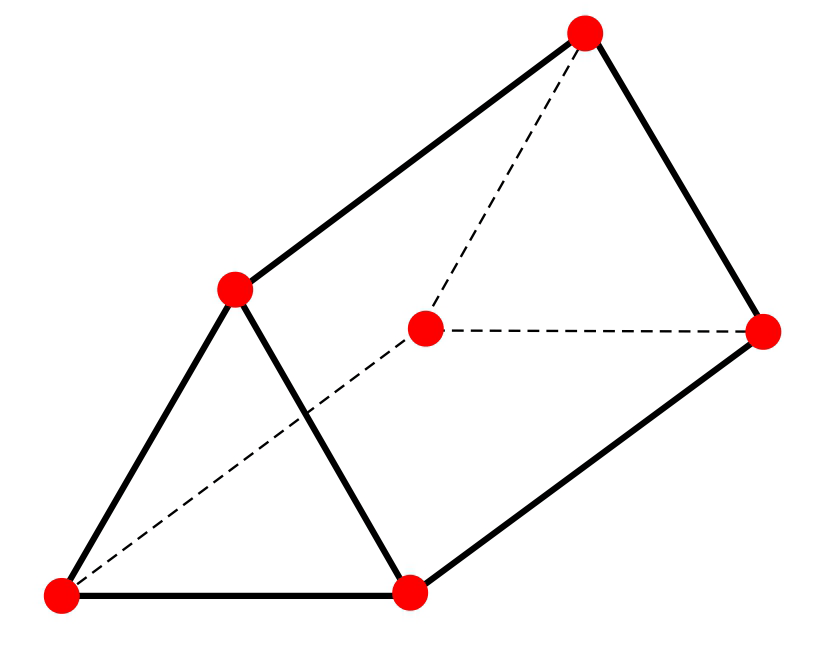Question #5:

Shawn has an 8-sided die for a game he plays with his friends. How many edges does the die have?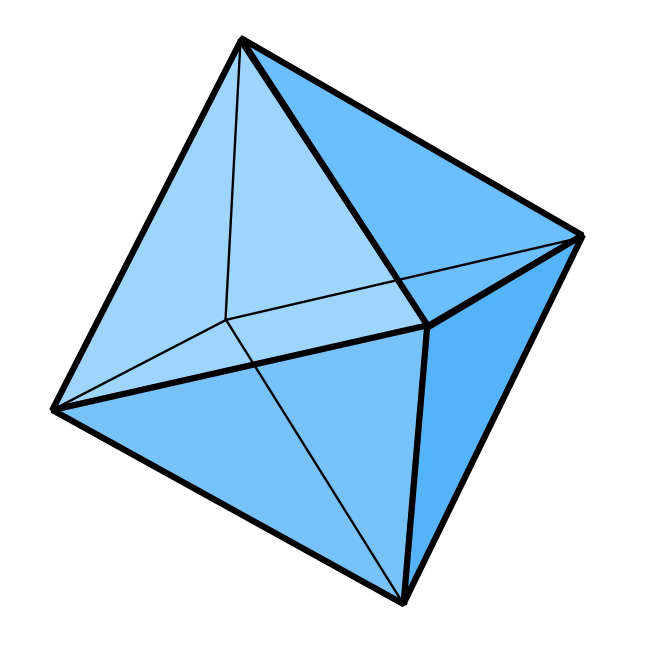8 edges

10 edges

12 edges

14 edges